International Tables for Crystallography (2006). Vol. C. ch. 1.2, pp. 6-9https://doi.org/10.1107/97809553602060000573

## Contents

• 1.2. Application to the crystal systems  (pp. 6-9)
• 1.2.1. Triclinic crystal system  (p. 6) | html | pdf |
• 1.2.2. Monoclinic crystal system  (p. 6) | html | pdf |
• 1.2.2.1. Setting with `unique axis b '  (p. 6) | html | pdf |
• 1.2.2.2. Setting with `unique axis c '  (p. 6) | html | pdf |
• 1.2.3. Orthorhombic crystal system  (pp. 6-7) | html | pdf |
• 1.2.4. Tetragonal crystal system  (p. 7) | html | pdf |
• 1.2.5. Trigonal and hexagonal crystal system  (pp. 7-9) | html | pdf |
• 1.2.5.1. Description referred to hexagonal axes  (pp. 7-8) | html | pdf |
• 1.2.5.2. Description referred to rhombohedral axes  (pp. 8-9) | html | pdf |
• 1.2.6. Cubic crystal system  (p. 9) | html | pdf |
• References | html | pdf |
• Tables
• Table 1.2.4.1. Assignment of integers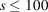to pairs h , k with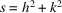(p. 7) | html | pdf |
• Table 1.2.5.1. Assignment of integersto pairs h , k with(p. 8) | html | pdf |
• Table 1.2.5.2. Assignment of integers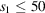to triplets h , k , l with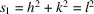and to integers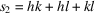(p. 8) | html | pdf |
• Table 1.2.6.1. Assignment of integersto triplets h , k , l with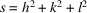(p. 9) | html | pdf |